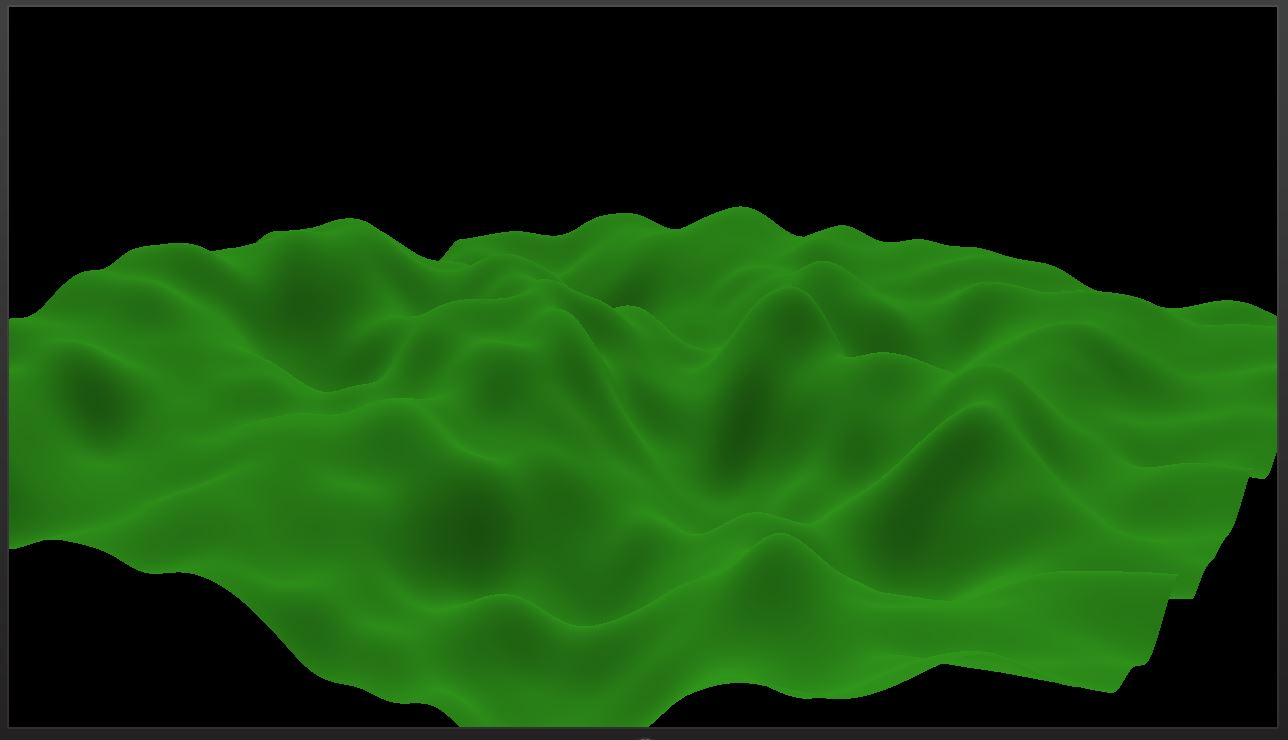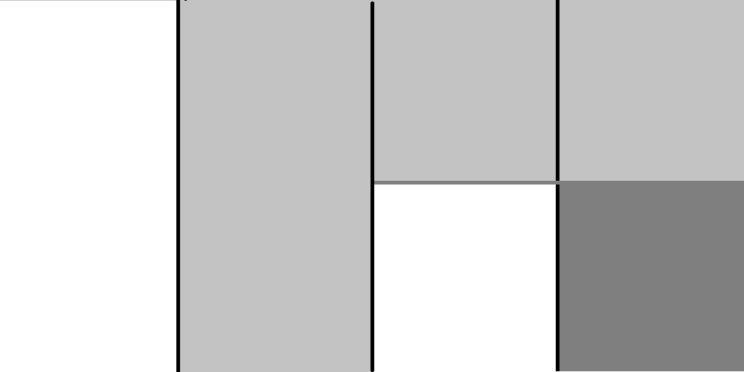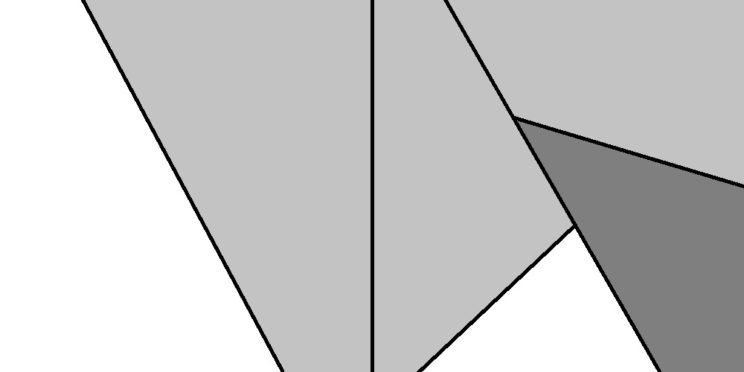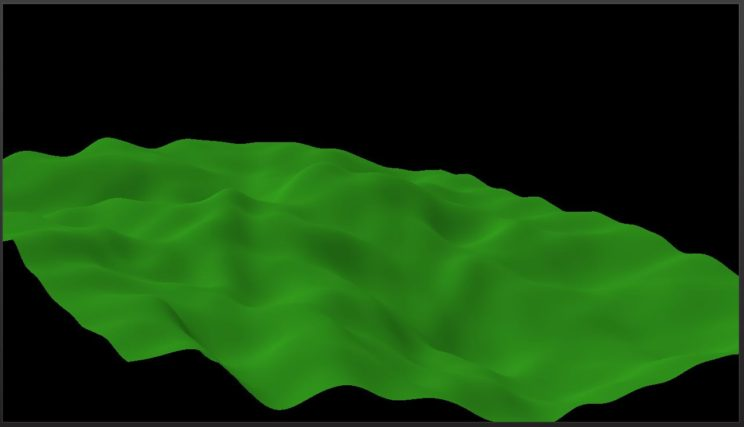# HoloLens Terrain Generation Demo Part 4 – Fault Formation

To generate my terrain, I’m going to use a variation on a fairly old algorithm. The earliest article I know of describing Fault Formation was published in 1994. Here’s another good description of it with some variations.
The idea behind the algorithm is to simulate the land shaping processes inherent in plate tectonics. As plates move, they grind together and, in some cases, one plate will slide under the other. This causes one side of the fault to lift up and the other to sink. While there are other types of faults, it is this specific type (reverse faults) that the Fault Formation algorithm seeks to simulate.

I’ll refer you to the Lighthouse 3D description for images of the basic Fault Formation algorithm, rather than replicating them here. The general idea is straight forward. Each iteration, randomly place a fault line across your height map. Any pixels on the right side of the line get raised, while any on the left get lowered. There are many possible ways to choose how much to raise or lower those pixels. Lighthouse provides a number of possible solutions, including using a small constant value, while Robert Krten suggests using a value that changes linearly based on the number of iterations. The former method would require more iterations, while the latter would require more smoothing of the final result. The smoothing of the terrain can also be performed in different ways. In his article, Mr Krten1 uses a simplified FIR Filter per iteration. I find if you apply the filter after you’ve built up the terrain, you will need fewer iteration of the filter, saving cycles. You could also use a Gaussian blur or similar. And for perhaps the best but slowest results, you could implement an actual erosion simulation.

For this project, I’m using a variation of the Fault Formation algorithm that I came up with a few years ago. The idea behind this variation was to provide a bit more structure and control to the Fault Formation algorithm. I’m not entirely sure I succeeded in making a ‘better’ algorithm. It is slower, but has more variables you can set to control the outcome; but the outcome still seems pretty random. Still, I think it’s worth playing with.
My algorithm uses a Binary Space Partitioning (BSP) Tree at each iteration to generate a tree of faults. Then, for each pixel of the height map, we walk the tree to determine the final height of the pixel. I start with a base height value and halve that value as I move down the tree.
The simple form of this algorithm is to generate each fault in the tree separately, as if it exists on its own. The nature of the tree structure will ensure that height map pixels will only be affected by certain faults.
Here’s the BSP tree definition I’m using:

class BSPNode {
public:
BSPNode();
// Constructor to create new Node with supplied parent node.
BSPNode(BSPNode *parent);
~BSPNode();
void SetStartPos(float x, float y) { m_posStart.x = x; m_posStart.y = y; }
void SetStartPos(DirectX::XMFLOAT2 p) { m_posStart = p; }
void SetEndPos(float x, float y) { m_posEnd.x = x; m_posEnd.y = y; }
void SetEndPos(DirectX::XMFLOAT2 p) { m_posEnd = p; }
DirectX::XMFLOAT2 GetStartPos() { return m_posStart; }
DirectX::XMFLOAT2 GetEndPos() {	return m_posEnd; }
BSPNode *GetLeftChild() { return m_childLeft; }
BSPNode *GetRightChild() { return m_childRight; }
BSPNode *GetParent() { return m_parent; }
// Create the left child and return a pointer to it;
BSPNode *CreateLeftChild();
// Create the right child and return a pointer to it;
BSPNode *CreateRightChild();

private:
BSPNode*			m_childLeft;	// Left child node.
BSPNode*			m_childRight;	// Right child node.
BSPNode*			m_parent;		// The parent of this node.
DirectX::XMFLOAT2	m_posStart;		// Position of starting point of line segment representing line of the node.
DirectX::XMFLOAT2	m_posEnd;		// Position of the end point of the line segment representing line of the node.
};

And here’s the function to build the BSP tree:

void Terrain::BuildBSPTree(BSPNode* current, unsigned int depth) {
unsigned int h = m_hHeightmap * m_resHeightmap + 1;
unsigned int w = m_wHeightmap * m_resHeightmap + 1;
std::uniform_int_distribution<int> distX(0, w);
std::uniform_int_distribution<int> distY(0, h);

current->SetStartPos(distX(generator), distY(generator));
current->SetEndPos(distX(generator), distY(generator));

if (depth <= 1) return;

BuildBSPTree(current->CreateLeftChild(), depth - 1);
BuildBSPTree(current->CreateRightChild(), depth - 1);
}

The function that performs an iteration and updates the height map:

void Terrain::IterateFaultFormation(unsigned int treeDepth, float treeAmplitude) {
BSPNode root;
BuildBSPTree(&root, treeDepth);

// for each point in the height map, walk the BSP Tree to determine height of the point.
for (unsigned int y = 0; y < m_hHeightmap * m_resHeightmap + 1; ++y) {
for (unsigned int x = 0; x < m_wHeightmap * m_resHeightmap + 1; ++x) {
BSPNode* current = &root;
float amp = treeAmplitude;
float h = 0;

for (unsigned int d = 1; d <= treeDepth; ++d) {
auto start = current->GetStartPos();
auto end = current->GetEndPos();
float dx = end.x - start.x;
float dy = end.y - start.y;
float ddx = x - start.x;
float ddy = y - start.y;

if (ddx * dy - dx * ddy > 0) {
current = current->GetRightChild();
h += amp;
} else {
current = current->GetLeftChild();
h -= amp;
}

amp /= 2.0f;
}

m_heightmap[y * (m_wHeightmap * m_resHeightmap + 1) + x] += h;
}
}
}

The down side of this method is that the generated terrain is more random and appears to be less structured than the original Fault Formation Algorithm. This is caused by the fact that different pixels are affected by different faults. You will also likely run into situations where all of the pixels affected by a certain fault will be on the same side of the fault. This isn’t necessarily an issue, but wasn’t really what I wanted when I was thinking about this algorithm.As you can see from the above image, this algorithm doesn’t appear to have any rhyme or reason to any given iteration. Building up a terrain from this doesn’t look terrible, though.

Perhaps unintuitively, I came up with the simplified version of the algorithm after coming up with a more complicated version first. When I was originally experimenting with this idea, I started with what was basically a quad tree. I divided the height map using fault lines that exactly cut the height map in half.That would clearly make for a pretty boring height map. I added randomness back in by randomly choosing the initial line, then randomly selecting a starting point along that line for each child line. Then I randomly determined an angle off of that line to project from.Making the tree deeper, we get a more interesting structure:

The code for this version is, unsurprisingly, quite a bit more complex:

// Perform intersection test between two line segments
// take in 4 points. Make p1 and p2 the current line segment. Make p3 and p4 the one to intersect against.
// Return A: The point of intersection. Pass by reference.
// return j: j = ua. How far along the current line the intersection happens. Pass by reference.
// return k: k = ub. How far along the intersecting line the intersection happens. Pass by reference.
// return boolean value. True if intersection happens, false if it doesn't.
bool Intersect(float px1, float py1, float px2, float py2, float px3, float py3, float px4, float py4,
float &ax, float &ay, float &j, float &k) {
float denom = (py4 - py3) * (px2 - px1) - (px4 - px3) * (py2 - py1);

if (denom == 0) { // then the two lines are parallel and will never intersect.
ax = ay = j = k = 0;
return false;
}

float ua = ((px4 - px3) * (py1 - py3) - (py4 - py3) * (px1 - px3)) / denom;
float ub = ((px2 - px1) * (py1 - py3) - (py2 - py1) * (px1 - px3)) / denom;
j = ua;
k = ub;

ax = px1 + ua * (px2 - px1);
ay = py1 + ua * (py2 - py1);

return true;
}

void Terrain::BuildBSPTree(BSPNode *current, unsigned int depth) {
unsigned int h = m_hHeightmap * m_resHeightmap + 1;
unsigned int w = m_wHeightmap * m_resHeightmap + 1;
std::uniform_int_distribution<int> distX(0, w);
std::uniform_int_distribution<int> distY(0, h);
std::uniform_real_distribution<float> distL(0.0f, 1.0f);
std::uniform_real_distribution<float> distT(-45.0f, 45.0f);

BSPNode* parent = current->GetParent();
if (parent) {
auto start = parent->GetStartPos();
auto end = parent->GetEndPos();
float m = (end.y - start.y) / (end.x - start.x); // find the slope of the parent line.
float b = start.y - m * start.x; // find b for the equation y = mx + b by solving for b using the start point b = y - mx.
float dx = end.x - start.x;
float qdx = dx / 4;
float hdx = dx / 2;

// find random start point along parent line, somewhere between 0.25 and 0.75 along the segment.
float x = start.x + qdx + distL(generator) * hdx;
float y = m * x + b;
current->SetStartPos(x, y);

// find random direction off of point and look for end point. Line will either intersect with the border of the terrain, or it will intersect with an ancestor.
// we want the random direction off of our child's start point to be somewhere between 45 and 135 degrees from the parent line.
// we can find the perpendicular left vector as our direction (e - s) expressed as (x, y) inverted to (-y, x)
// we can find the perpendicular right vector as our direction (e - s) expressed as (x, y) inverted to (y, -x)
float lx, ly;
// figure out if this is the left or right child.
if (current == parent->GetLeftChild()) {
lx = -1 * (end.y - start.y);
ly = end.x - start.x;
}
else {
lx = end.y - start.y;
ly = -1 * (end.x - start.x);
}

// Now randomly choose a value between -45 degrees and 45 degrees (expressed in radians).
// Now rotate our perpendicular direction vector by theta
float llx = lx * cos(theta) - ly * sin(theta);
float lly = lx * sin(theta) + ly * cos(theta);

// Now find the end point by intersecting first with the borders of the map and then with ancestors.
float x2 = x + llx;
float y2 = y + lly;
// first the bottom border (0,0) to (MAPSIZE - 1, 0). Since this is the first, if it intersects at all with ua > 0, A will become
// our new end point to test against.
float x3 = 0;
float y3 = 0;
float x4 = w - 1;
float y4 = 0;
float ua, ub, ax, ay;
bool in = Intersect(x, y, x2, y2, x3, y3, x4, y4, ax, ay, ua, ub);
if (in && (ua > 0)) { // we don't want to use it if in is false
x2 = ax;
y2 = ay;
}
// test against right border (MAPSIZE - 1, 0) to (MAPSIZE - 1, MAPSIZE - 1). Be more picky. 0 < ua < 1 and 0 < ub < 1.
x3 = x4;
y3 = y4;
y4 = w - 1;
in = Intersect(x, y, x2, y2, x3, y3, x4, y4, ax, ay, ua, ub);
if (in && (ua > 0) && (ua < 1) && (ub > 0) && (ub < 1)) {
x2 = ax;
y2 = ay;
}
// test against top border (MAPSIZE - 1, MAPSIZE - 1) to (0, MAPSIZE - 1).
y3 = y4;
x4 = 0;
in = Intersect(x, y, x2, y2, x3, y3, x4, y4, ax, ay, ua, ub);
if (in && (ua > 0) && (ua < 1) && (ub > 0) && (ub < 1)) {
x2 = ax;
y2 = ay;
}
// test against left border (0, MAPSIZE - 1) to (0, 0)
x3 = x4;
y4 = 0;
in = Intersect(x, y, x2, y2, x3, y3, x4, y4, ax, ay, ua, ub);
if (in && (ua > 0) && (ua < 1) && (ub > 0) && (ub < 1)) {
x2 = ax;
y2 = ay;
}
// now that we know where the line segment intersects the border of the map, we need to see if it intersects an ancestor before that happens.
// It cannot intersect its immediate parent anywhere but at the start point which we already have so ignore the parent and move on to the
// grandparent.
parent = parent->GetParent();
while (parent) { // This will kick out once the ancestor is NULL
auto p3 = parent->GetStartPos();
auto p4 = parent->GetEndPos();
in = Intersect(x, y, x2, y2, p3.x, p3.y, p4.x, p4.y, ax, ay, ua, ub);
if (in && (ua > 0) && (ua < 1) && (ub > 0) && (ub < 1)) {
x2 = ax;
y2 = ay;
}

parent = parent->GetParent();
}
current->SetEndPos(x2, y2);
} else {
// if this is the root node, we just randomly set the initial divider.
current->SetStartPos(distX(generator), distY(generator));
current->SetEndPos(distX(generator), distY(generator));
}

if (depth <= 1) return;

BuildBSPTree(current->CreateLeftChild(), depth - 1);
BuildBSPTree(current->CreateRightChild(), depth - 1);
}

At this point, we have a decent enough terrain to use for this project, and we have a few variables we can use to control the final terrain. At this point, we haven’t smoothed the terrain at all, so it is quite rough. For this project, I don’t want to bother with a more complex implementation, so I’m just going to use Robert Krten’s FIR filter. I’m just going to apply a few iterations after generating the terrain.
*EDIT* According to Shawn Stevenson, who posted below in the comments, the filter I am presenting here is actually an IIR Filter. I’m still trying to determine for sure as I don’t know very much about Digital Signal Processing and took this code from a different source.
My source was Trent Polak’s Focus on 3D Terrain Programming. He cites Jason Shankel’s article ‘Fractal Terrain Generation – Fault Formation’ from Game Programming Gems. The implementation is slightly different than Robert Krten’s own, but I believe the final output is the same.
I will update this post once I am certain what sort of filter this actually is.
*EDIT 2* I took a closer look at Shawn Stevenson’s FIR filter tutorial and I’m now pretty certain this code is indeed a FIR filter. It is simplified compared to what he presents, but it does appear to follow the same formula.
He presents the equation y(n)=\sum_{k=0}^{N-1}h(k)x(n-k) where x() is the input and h() is a list of filter coefficients. He then presents a generic function capable of handling any number of coefficients. The version that we are using, passed down to us from the sources listed above, is a very specific case.
In our case, N = 2 and the coefficients are k and 1 – k (where in our code filter is k).
One difference between this implementation and the definition of a FIR filter provided by Shawn is that his definition states that the output of the filter depends only on the inputs and not on previous outputs. If you look at the below code, you’ll note that at each iteration of each loop we are using the previous iteration’s output as input to the current pass. Based on Shawn’s definition, we should be doing something like the following:

for (int x = 1; x < w; ++x) {
float tempprev = m_heightmap[x + y * w];
m_heightmap[x + y * w] = filter * prev + (1 - filter) * m_heightmap[x + y * w];
prev = tempprev;
}

This change would allow our output to be dependent only on the input. This is a pretty minor change and would not likely impact the final result significantly, so I haven’t bothered to implement it in my code.
*EDIT 3* Barbarian has clarified in the comments that the version of the filter I am using is an IIR filter BECAUSE I am using the previous pass’ output as input for the current pass. I’m still happy with the results, but I’ll have to change the method name the next time I’m working on the code.
*End of Edits*

void Terrain::FIRFilter(float filter) {
unsigned int h = m_hHeightmap * m_resHeightmap + 1;
unsigned int w = m_wHeightmap * m_resHeightmap + 1;
float prev;

for (int y = 0; y < h; ++y) {
prev = m_heightmap[y * w];
for (int x = 1; x < w; ++x) {
prev = m_heightmap[x + y * w] = filter * prev + (1 - filter) * m_heightmap[x + y * w];
}

prev = m_heightmap[(w - 1) + y * w];
for (int x = w - 2; x >= 0; --x) {
prev = m_heightmap[x + y * w] = filter * prev + (1 - filter) * m_heightmap[x + y * w];
}
}

for (int x = 0; x < w; ++x) {
prev = m_heightmap[x];
for (int y = 1; y < h; ++y) {
prev = m_heightmap[x + y * w] = filter * prev + (1 - filter) * m_heightmap[x + y * w];
}

prev = m_heightmap[x + w * (h - 1)];
for (int y = h - 2; y >= 0; --y) {
prev = m_heightmap[x + y * w] = filter * prev + (1 - filter) * m_heightmap[x + y * w];
}
}
}100 iterations of BSP Tree Fault Formation + 20 iterations of FIR Filter with a filter value of 0.2f.

While I’ll likely mess around with the variables, and I would love to build a simple interface to change the variables interactively, I think this is ultimately the terrain generation system I’m going to stick with. Currently, we’re just running all of the iterations at start up. What I want to do next is change it so that we run one iteration per frame so we can see the terrain uplift as an animation. For next post, I’d also like to see if I can make it so we can reset the terrain with some sort of simple interaction from the user. Perhaps a basic click or gesture.